ď»ż Unit Circle

# Unit Circle

Top  Previous  Next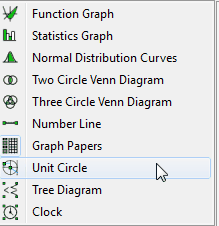The unit circle tool lets you draw unit circles showing the sine, cosine, tangent and cotangent ratios.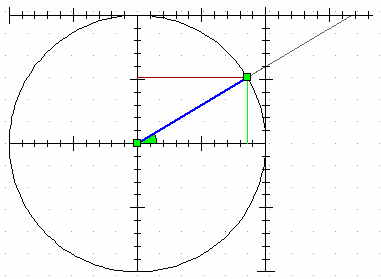Drawing a unit circle is identical to drawing a centre/radius circle.

Unit circle properties allow you to turn each ratio on or off - as well as defining how each ratio line is shown.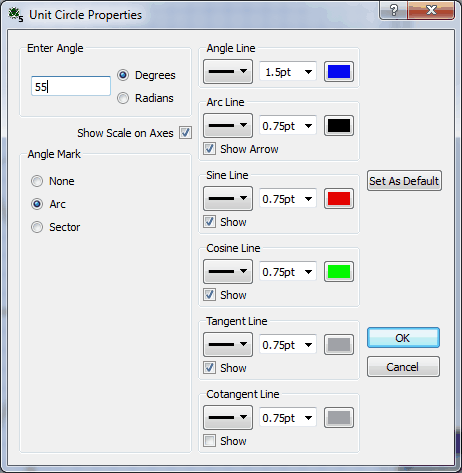You can change the angle by moving the control points at each end of the angle or by explicitly entering the angle in the screen above.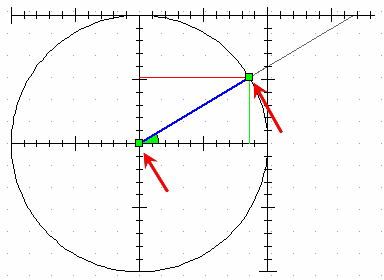Important Points

 • The unit circle can show positive or negative angles.  To get a negative angle, or an angle greater than 360 degrees, explicitly set the angle or use the keyboard commands.
 • Angles set by moving the control point with the mouse will always be between 0 and 360 degrees.
 • Explicit angles in radians can be entered in terms of pi.  For example, type 3pi/4.
 • Angles greater than 360 degrees will be show with an arc with multiple rotations.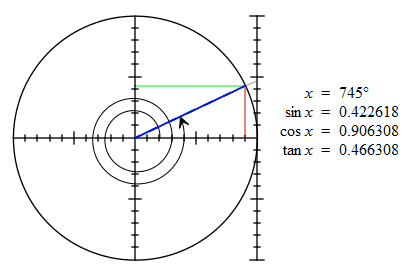Keyboard Commands

For demonstration purposes, the unit circle's angle can be controlled using the keyboard.

Home                        Resets the angle to 0°.

Up Arrow                Increases angle by 1°.

Down Arrow                Decreases angle by 1°.

Page Up                Increase angle by 5°.

Page Down                Decreases angle by 5°.

Available Parameters for Unit Circles

\$angle                        Angle expressed as the current angle type (degrees or radians)

\$degrees                Angle in degrees

\$radians                Angle in radians.  Angle will be show as a multiple of pi if possible.

\$sin

\$cos

\$tan

\$cot